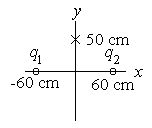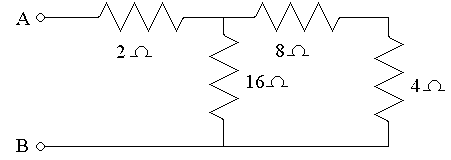First Name: ___________________ Last Name: ____________________ Section: _________

February 18, 2000 Physics 208

Exam 1

Print your name and section clearly on all five pages. (If you do not know your section number, write your TAs name.) Show all work in the space immediately below each problem. Your final answer must be placed in the box provided. Problems will be graded on reasoning and intermediate steps as well as on the final answer. Be sure to include units wherever necessary, and the direction of vectors. Each problem is worth 25 points. In doing the problems, try to be neat. Check your answers to see that they have the correct dimensions (units) and are the right order of magnitudes. You are allowed one 8½ x 11" sheet of notes and no other references. The exam lasts exactly 50 minutes.

(Do not write below)

SCORE:

Problem 1: __________

Problem 2: __________

Problem 3: __________

Problem 4: __________

TOTAL: ___________

First Name: ___________________ Last Name: ____________________ Section: _________

1. A point charge q1 is located at x = -60 cm and a point charge q2 is located at x = +60 cm on the x-axis.a. If q1 = -q2 = 7x10-9 C, what is the electric field at y = +50 cm on the y-axis? (10 pts.)

b. If q1 = +q2 = 7x10-9 C, what is potential (relative to infinity) at y = +50 cm on the y-axis? (10 pts.)

c. If q1 = -q2 = 7x10-9 C, what is the force between the charges? (5 pts.)

First Name: ___________________ Last Name: ____________________ Section: _________

1. A long, cylindrical conductor (radius = 1.0 mm) carries a charge of 4x10-12 C/m and is inside a second coaxial, hollow, cylindrical conductor (inner radius = 3.0 mm, outer radius = 4.0 mm) that has a charge of 8x10-12 C/m.
a. What is the electric field 2.0 mm from the axis of the conductors? (15 pts.)

b. What is the electric field 5.0 mm from the axis of the conductors? (10 pts.)

First Name: ___________________ Last Name: ____________________ Section: _________

1. A 20-?F capacitor charged to 2 kV and a 40-?F capacitor charged to 3 kV are suddenly connected to each other with the positive plate of each connected to the negative plate of the other.

2.

a. What total energy is stored in the capacitors before they are connected? (6 pts.)

b. What is the charge on the 20-?F capacitor after the two are connected? (7 pts.)

c. What is the voltage on the capacitors after they are connected? (6 pts.)

d. What is the total energy stored in the capacitors after they are connected? (6 pts.)

First Name: ___________________ Last Name: ____________________ Section: _________

3. In the circuit below, a current of 1.4 A flows in the 4-? resistor.a. What is the equivalent resistance between points A and B? (10 pts.)

b. What is the magnitude of the potential difference VA - VB? (10 pts.)

c. What total power is dissipated by the resistors? (5 pts.)